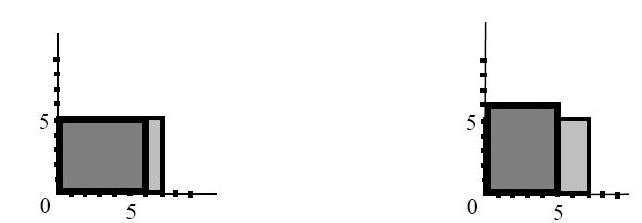Online JudgeProblem SetAuthorsOnline ContestsUser
Web Board
F.A.Qs
Statistical Charts
Problems
Submit Problem
Online Status
Prob.ID:
Register
Authors ranklist
Current Contest
Past Contests
Scheduled Contests
Award Contest
Register

Language:
Maximum Area Covered by Rectangles
 Time Limit: 2000MS Memory Limit: 65536K Total Submissions: 1193 Accepted: 487

Description

Once upon a time, there was a small kingdom in a small island. The king needed to give rewards to a general for his effort of fighting the enemy. The king was famous of his not being generous. So, the king gave a map of one 10000*10000 green rectangle to the general. (We say that the width of the rectangle is the side that is not longer than the other side. The other side is called the height. Therefore, a 3 * 4 rectangle has width 3 and height 4.) The general was given at most 100 sets of blue rectangles on the table. Each set contains at least 2 rectangles and at most 15 rectangles, and the width of rectangles in a set is the same. We also know that if rectangle A and rectangle B are in two different sets, then rectangle A does not contain rectangle B and rectangle B does not contain rectangle A. (Note that a rectangle has 4 boundary lines. Two lines in a plane are aligned if there is one line that contains both lines. Rectangle A contains rectangle B if you can completely place A on the top of B such that at least one boundary line of A is aligned with a boundary line of B and no parts of B is visible assuming rectangles are solid and non-transparent. For example, a 5*7 rectangle contains a 4*6 rectangle, but a 5*5 rectangle does not contain a 4 * 6 rectangle.) The total number of the blue rectangles is at most 1000.

The general could pick up as many blue rectangles as he wanted from the table and put them on the top of green rectangle. Each blue rectangle must have one corner sitting at the left-lower corner of the green rectangle,and one of the blue rectangle's boundary line is aligned with a boundary line of the green rectangle.

Area of the map covered by the blue rectangles will be granted to the general. This looks like a difficult task for the general. Fortunately, the general has a notebook computer. Please help the general to write a program to find out what is the maximum area the general can cover by those blue rectangles. You don't need to show how to put the blue rectangles. The output is the maximum area the general will be granted. For example, if there are two blue rectangles with dimensions 5 * 7 and 5 * 6. These two rectangles belong to the same set The following figure shows two possible ways to place the two rectangles.On the left, the total covered area is 35. On the right, the total covered area is 40, which is the maximum area that you can cover.

Input

There are m test data, m <= 10. In each test data, there are at most 1000 rectangles. The first line in a data set contains the number of rectangles. Each rectangle in the data set is represented in a line xy, where x and y are the width and height of the corresponding rectangle, respectively. There is a blank between x and y. Note that x and y are positive integers that are at most 10000. End of the input file is a single line of '-1'.

Output

The output should have m numbers in m lines. Each line reports the maximum covered area for the corresponding data set .

Sample Input

```2
5 7
5 6
-1```

Sample Output

`40`

Source

[Submit]   [Go Back]   [Status]   [Discuss]Home PageGo BackTo top